# Equilibrium constant

Equilibrium: Equilibrium constant

The equilibrium constant (K) is a number that represents the concentrations of reactants and products of a reversible reaction at a given temperature

K = [products]/[reactants]

For a general chemical equation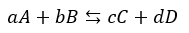The equilibrium constant is written as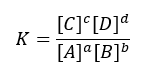Where:

• Small superscript letters are the stoichiometry coefficients
• [A] is the concentration chemical species where A relative to standard state
• The concentration of solutes should be expressed as moles per litre (M).
• Concentrations of gases should be expressed in barsas Pgas, emphasize pressure instead of concentration where 1 bar = 105 Pa; 1 atm = 1.01325 bar
• Concentrations of pure solids, pure liquids and solvents are omitted since they are unity and remains constant.

Note that, a reaction is favoured when K >1. If the equilibrium constant is a high number, the reaction favours the products; the concentration of the products is greater than that of the reactants. Also, K has no units, dimensionless.The larger the value for the equilibrium constant the more the reaction goes to completion.

The equilibrium constant for a net reaction that consists of two or more steps is the product of the equilibrium constants for the individual steps. As shown in the figure below, the equilibrium constant can be manipulated depending on the direction of the reaction. Incidentally, The equilibrium constant of a reaction in the reverse reaction is the reciprocal of the equilibrium constant of the forward reaction. The ratio of the rate constants is a constant (as long as T is constant).Note: Temperature is same for both reaction.

Figure 1:Manipulation of equilibrium constant expressions.

The equilibrium constant can be expressed in terms of the concentration as well as the pressure of the constituents such as in the case of gases. The pressure is proportional to concentration for gases in a closed system, the equilibrium expression can also be written with respect to pressure.

The relationship between Kc and Kp can be explained using the universal gas law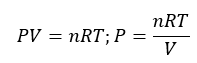Plugging this equation into the expression for Kp for each substance, the relationship between Kc and Kp can be shown as

Kp= Kc(RT)n

Where ∆n = (moles of gaseous product) − (moles of gaseous reactant)

Consider the reaction:

2SO2(g) + O2(g) ⇄ 2SO3(g)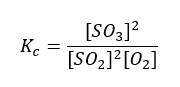It may also be written as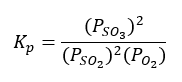Assuming ideal gas behaviour

• where PV = nRT and P = (n/V)RT = [M]RT
• and PSO3 = [SO3]RT; PSO2 = [SO2]RT; PO2 = [O2]RT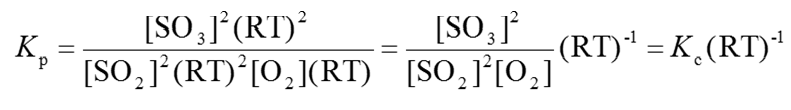A homogeneous equilibrium is one in which all reactants and products are in the same phase while a heterogeneous equilibriumis one in which all reactants and products are not in the same phase.

In general, Kp = Kc(RT)∆n and ∆n = (c+d)-(a+b) and in heterogeneous systems, only the coefficients of the gaseous species are counted.

For other reactions:

• 2NO2(g) ⇄ N2O4(g); Kp = Kc(RT)-1
• H2(g) + I2(g)⇄ 2 HI(g); Kp = Kc
• N2(g) + 3H2(g)⇄ 2 NH3(g); Kp = Kc(RT)-2